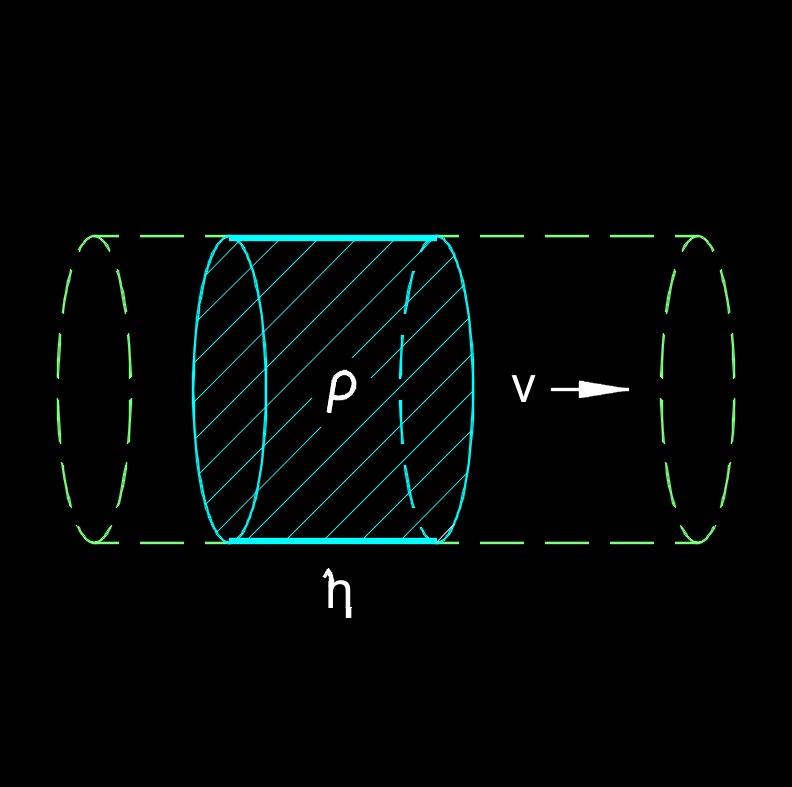# Viscosity

Written by Jerry Ratzlaff on . Posted in Fluid DynamicsViscosity, abbreviated as $$\eta$$ (Greek symbol eta), is the measure of the internal friction/resistance to the flow of a liquid.  Lower viscosity fluids flow easily in pipes where high viscosity fluids have a have a higher pressure drop.  Viscosity of fluids is typically temperature dependent and is not affected as dramatically by pressure as gas viscosity.  In fact, typically as a liquid temperature increases, the velocity decreases.  When a gas temperature increases, the viscosity increases!

There are two different types of viscosity, dynamic viscosity and kinematic viscosity.  Higher the viscosity the more resistance to flow.  Lower the viscosity the less resistance to flow.  Temperature also plays a part, lower the temperature, higher the resistance.  Higher the temperature, lower the resistance.  A Viscometer meter can be used to measure viscosity.

## Viscosity formula

 $$\large{ \eta = \frac { g \; d^2\; \left( \rho_p \;-\; \rho_m \right) } {18\; v} }$$ (Stokes' law)

### Where:

$$\large{ \eta }$$  (Greek symbol eta) = viscosity of medium

$$\large{ \rho_m }$$ = density of medium

$$\large{ \rho_p }$$ = particle density

$$\large{ d }$$ = diameter

$$\large{ g }$$ = gravitational acceleration

$$\large{ v }$$ = velocity

## Viscosity Index

Viscosity index, abbreviated as VI, is a measure of a fluid's sensitivity to change in viscosity with change in temperature.

### Viscosity Index Standards

• ASTM Standards
• ASTM D2116 - Standard Method for Conversion of Kinematic Viscosity to Saybolt Universal Viscosity or to Saybolt Furol Viscosity
• ASTM D2270 - Standard Practice for Calculating Viscosity Index from Kinematic Viscosity at 40 and 100°C
• ASTM STP168 - ASTM Viscosity Index Calculated from Kinematic Viscosity
• ASTM STP532 - Viscosity Testing of Asphalt and Experience with Viscosity Graded Specifications